##### The Japanese Bonsai specialist
Direct order Contact Help / Services Newsletter# Juniperus chinensis itoigawa ref 16090191

Juniperus chinensis itoigawa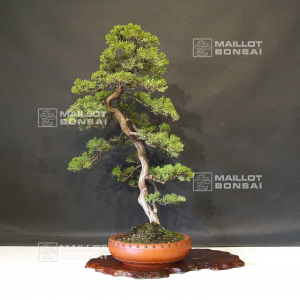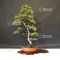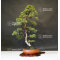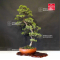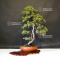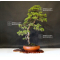ref. : 10306

1.880,00

voluminous/heavy item extra shipping of 12,00

Available quantity : 1Order

###### Description

Important master piece.

Height of the tree without its pot: 800 mm. Width of the branches: 510 mm.

Artisanal non-enamelled pot with patina, signed Suishoen, from Tokoname, Japan: 310*90 mm, brick color.

Sokan/bunjin-ji style. Formed and ramified trays.

Diameter of the trunk at its base: about 25/35 mm.

The bark of this tree is perfectly formed because it is more than 35 years old.

It comes from yamadori and was cultivated in pot at Master Tomoya Nishikawa's home for 20 years, in Himeji, Japan. Presence of jins.

Not any strong wire wound nor cutting wound.

Remarkable subject! For strict collector. Admire the movement of the trunk with the entirely natural shari (do not mistake with tanukis), and the beauty of the small trunk that enhance the biggest. Itoigawa variety with thin green canopy scales. Photographed in October 2019, shelf non-included.

#with 4.4 #itoigawa 3.8 #trunk 3.5 #chinensis 2.9 #juniperus 2.9 #outdoor 2.7 #bonsai 2.6 #formed 2.6 #master 2.6 #years 2.5

Formule
(( ROUND((CHAR_LENGTH(b.article_nom)-CHAR_LENGTH(REPLACE(b.article_nom, 'with', '')))/LENGTH('with')) + ROUND((CHAR_LENGTH(b.article_description)-CHAR_LENGTH(REPLACE(b.article_description, 'with', '')))/LENGTH('with')) ) * 4.4) + (( ROUND((CHAR_LENGTH(b.article_nom)-CHAR_LENGTH(REPLACE(b.article_nom, 'itoigawa', '')))/LENGTH('itoigawa')) + ROUND((CHAR_LENGTH(b.article_description)-CHAR_LENGTH(REPLACE(b.article_description, 'itoigawa', '')))/LENGTH('itoigawa')) ) * 3.8) + (( ROUND((CHAR_LENGTH(b.article_nom)-CHAR_LENGTH(REPLACE(b.article_nom, 'trunk', '')))/LENGTH('trunk')) + ROUND((CHAR_LENGTH(b.article_description)-CHAR_LENGTH(REPLACE(b.article_description, 'trunk', '')))/LENGTH('trunk')) ) * 3.5) + (( ROUND((CHAR_LENGTH(b.article_nom)-CHAR_LENGTH(REPLACE(b.article_nom, 'juniperus', '')))/LENGTH('juniperus')) + ROUND((CHAR_LENGTH(b.article_description)-CHAR_LENGTH(REPLACE(b.article_description, 'juniperus', '')))/LENGTH('juniperus')) ) * 2.9) + (( ROUND((CHAR_LENGTH(b.article_nom)-CHAR_LENGTH(REPLACE(b.article_nom, 'chinensis', '')))/LENGTH('chinensis')) + ROUND((CHAR_LENGTH(b.article_description)-CHAR_LENGTH(REPLACE(b.article_description, 'chinensis', '')))/LENGTH('chinensis')) ) * 2.9) + (( ROUND((CHAR_LENGTH(b.article_nom)-CHAR_LENGTH(REPLACE(b.article_nom, 'master', '')))/LENGTH('master')) + ROUND((CHAR_LENGTH(b.article_description)-CHAR_LENGTH(REPLACE(b.article_description, 'master', '')))/LENGTH('master')) ) * 2.6) + (( ROUND((CHAR_LENGTH(b.article_nom)-CHAR_LENGTH(REPLACE(b.article_nom, 'formed', '')))/LENGTH('formed')) + ROUND((CHAR_LENGTH(b.article_description)-CHAR_LENGTH(REPLACE(b.article_description, 'formed', '')))/LENGTH('formed')) ) * 2.6) + (( ROUND((CHAR_LENGTH(b.article_nom)-CHAR_LENGTH(REPLACE(b.article_nom, 'years', '')))/LENGTH('years')) + ROUND((CHAR_LENGTH(b.article_description)-CHAR_LENGTH(REPLACE(b.article_description, 'years', '')))/LENGTH('years')) ) * 2.5) + (( ROUND((CHAR_LENGTH(b.article_nom)-CHAR_LENGTH(REPLACE(b.article_nom, 'japan', '')))/LENGTH('japan')) + ROUND((CHAR_LENGTH(b.article_description)-CHAR_LENGTH(REPLACE(b.article_description, 'japan', '')))/LENGTH('japan')) ) * 2.5) + (( ROUND((CHAR_LENGTH(b.article_nom)-CHAR_LENGTH(REPLACE(b.article_nom, 'wound', '')))/LENGTH('wound')) + ROUND((CHAR_LENGTH(b.article_description)-CHAR_LENGTH(REPLACE(b.article_description, 'wound', '')))/LENGTH('wound')) ) * 2.5)

## Secure payment## Delivery

Our logistic partners :